Journal of Vibroengineering

Published: 15 February 2019

# A fast-multi-pole accelerated method of fundamental solutions for 2-D broadband scattering of SH waves in an infinite half space

Zhongxian Liu1
Zhikun Wang2
Linping Guo3
Dong Wang4
Fengjiao Wu5
1, 2, 3, 4, 5Tianjin Key Laboratory of Civil Structure Protection and Reinforcement, Tianjin Chengjian University, Tianjin, China
1School of Engineering, University of Mississippi, Mississippi, USA
4Hebei Building Research Technical Co. Ltd, Shijiazhuang, China
Corresponding Author:
Zhongxian Liu
Views 100

#### Abstract

The traditional method of fundamental solution (T-MFS) is known as an effective method for solving the scattering of elastic waves, but the T-MFS is inefficient in solving large-scale or broadband frequency problems. Therefore, in order to improve the performance in efficiency and memory requirement for treating practical complex 2-D broadband scattering problems, a new algorithm of fast multi-pole accelerated method of fundamental solution (FM-MFS) is proposed. Taking the 2-D scattering of SH waves around irregular scatterers in an elastic half-space as an example, the implementation steps are presented in detail. Based on the accuracy and efficiency verification, the FM-MFS is applied to solve the broadband frequency scattering of plane SH waves around group cavities, inclusions, a V-shaped canyon and a semi-elliptical hill. It shows that, compared with T-MFS, the FM-MFS has great advantages in reducing the consumed CPU time and memory for 2-D broadband scattering. Besides, the FM-MFS has excellent adaptability both for broad-frequency and complex-shaped scattering problems.

## 1. Introduction

The scattering of elastic waves is an attractive and significant topic in many fields such as in earthquake engineering, geophysics, civil engineering, etc. In general, the calculation methods for the wave scattering include the analytical methods and numerical methods. The analytical methods are mainly referred to the wave function expansion methods [1-8]. The numerical methods include finite difference methods (FDM) [9, 10], finite element methods (FEM) [11, 12], boundary element methods (BEM) [13-20], and more works can be found in a review by Sanchez-Sesma et al.  and by Manolis and Dineva .

Among different boundary-type methods, the method of fundamental solution (MFS) not only reduces the dimension of problem and automatically satisfy the boundary conditions at infinite, but also is a meshless method. In the MFS, the approximate solution of the partial differential equation is constructed by the linear combination of a series of Green’s functions. In order to avoid singularities, the fictitious wave sources are placed at some distance to the physical boundaries of scatters. The densities of the fictitious sources are firstly obtained by the boundary integral equations discretized through boundary collocation points. So, the MFS can also be regarded as a special indirect boundary integral equation method which is very similar to the Trefftz method .

Due to the excellent numerical accuracy and simplicity of usage, the MFS (also named indirect boundary integral equation method) has been widely applied in the field of wave scattering in an elastic medium [24-32]. However, just as other boundary-type numerical methods, the MFS suffers a large difficulty in treating large-scale models. A significant weakness of MFS is that the calculation efficiency of solving high-DOF problem (such as large scale, broadband frequency or multi-body scattering) is seriously affected by its dense characteristic of the equation matrix. Chen et al.  greatly improved the computational efficiency of MFS using a sparse storage method, but the method still has some limitations to overcome this weakness. Some researchers tried to combine the FMM with the MFS in recent years. In essence, The FMM separates source points from collocation points of the kernel function expressions to change the Green’s function calculation from one-to-one interaction to group-to-group interaction. Then the FMM can substantially reduce the memory requirement and improve the computational efficiency [34-36]. The FM-MFS has been developed for solving many large-scale practical engineering problems [37-39]. The memory of MFS can be reduced to $O$ ($N$) by the latest development of FMM, and the computation amount is reduced to $O$ ($N\mathrm{l}\mathrm{o}\mathrm{g}2N$) order. It should be noted that the references mentioned above are all about the potential problems, the static analysis or the acoustic simulation. According to the author’s knowledge, the FM-MFS applied to elastic waves scattering problem in solid and the corresponding research are still rare at present. It is worth mentioned that there have been some reports related to the FMM-BEM [40, 41], but the associated BEM is largely different from the meshless MFS.

In this paper, in order to overcome the disadvantage of traditional MFS and solve large-scale elastic wave-motion problems in a half-space, a new algorithm of the FM-MFS is proposed and validated. The method is based on the single layer potential theory, and introduces the fast multi-pole expansion to construct the Green’s functions matrix. The virtual source density is obtained through GMRES iteration, of which the solving process is direct, and it is convenient for numerical implementation. Taking the 2-D scattering of SH waves around an irregular scatter in an elastic half-space as an example, the implementation steps are presented in detail, and then several typical examples indicate the excellent accuracy and efficiency of the method. Finally, the scattering of plane SH waves by randomly distributed cavity or inclusion group, by a canyon and hill topography in a half-space are solved and discussed.

Fig. 1 shows the calculation model. Assuming the incident waves are plane SH waves $\lambda$, $\mu$ are the two parameters of Lame constant, and $\rho$ is the medium density $k$ is the shear wave number, defined as $k=\omega /\beta$, where $\omega$ is the circular frequency of seismic waves, $\beta$ is the velocity of shear waves. The steady-state motion equation in isotropic homogeneous medium $D$ is as follows:

1
$\frac{{\partial }^{2}{u}_{z}}{\partial {x}^{2}}+\frac{{\partial }^{2}{u}_{z}}{\partial {y}^{2}}+{k}^{2}{u}_{z}=0,$

where ${u}_{z}$ is the anti-plane displacement. Note that the time factor $\mathrm{e}\mathrm{x}\mathrm{p}\left(\mathrm{i}\omega t\right)$ is omitted in Eq. (1) and thereafter.

According to numerical experience, the wave sources can be placed within 0.4-0.6 times of the relevant radius of the scatterer for low frequency waves (non-dimensional frequency $ka\le 2\pi$, $a$ is the scatterer radius); while for high frequency wave ($ka>2\pi$), the wave sources should better be placed within 0.7-0.9 times of the relevant radius of the scatterer.

Firstly, the wave field should be separated. And the total displacement field and the stress field in the elastic half-space can be expressed as:

2
${u}_{z}^{t}\left(x\right)={u}_{z}^{ff}\left(x\right)+{u}_{z}^{ss}\left(x\right),$
3
${\sigma }_{nz}^{t}\left(x\right)={\sigma }_{nz}^{ff}\left(x\right)+{\sigma }_{nz}^{ss}\left(x\right),$

where ${u}_{z}^{ff}$ and ${\sigma }_{nz}^{ff}$ are anti-plane displacement and shear stress of the free-field under incident plane SH waves. ${u}_{z}^{ss}$ and ${\sigma }_{nz}^{ss}$ are displacement and stress of the scattered wave field. The scattered field in $D$ is constructed by the virtual sources $S$. Consequently, the boundary condition can be written as:

4
${\sigma }_{nz}^{ss}\left(x\right)=-{\sigma }_{nz}^{ff}\left(x\right).$

According to the single layer potential theory, virtual sources on $S$ produce the scattered field in the half-space. Related displacement and stress are expressed as follows:

5
${u}_{z}^{ss}\left(x\right)={\int }_{s}{\varphi }_{z}\left(y\right){G}_{k}\left(x,y\right)dS,$
6
${\sigma }_{nz}^{ss}\left(x\right)={\int }_{s}{\varphi }_{z}\left(y\right){T}_{k}\left(x,y\right)dS.$

In which $x\in D$, $y\in S$. ${\varphi }_{z}\left(y\right)$ is the strength of shear-wave line source corresponding to $y$ position on virtual sources $S$. ${G}_{k}\left(x,y\right)$ and ${T}_{k}\left(x,y\right)$ are the displacement Green's functions and stress Green’s functions in the elastic half-space which can be introduced as:

7
${G}_{k}\left(x,y\right)=\frac{\mathrm{i}}{4}\left({H}_{0}^{\left(2\right)}\left(kr\right)+{H}_{0}^{\left(2\right)}\left(k{r}^{\mathrm{\text{'}}}\right)\right),$
8
${T}_{k}\left(x,y\right)=\mu \left(\frac{\partial {G}_{k}\left(x,y\right)}{\partial {n}_{x}}+\frac{\partial {G}_{k}\left(x,y\right)}{\partial {n}_{y}}\right),$

where ${H}_{0}^{\left(2\right)}\left(•\right)$ is the Hankel function of the second kind with order 0, ${n}_{x}$ and ${n}_{y}$ are the unit normal vectors, $r=\left|x-y\right|$, $r\mathrm{\text{'}}=\left|x-y\mathrm{\text{'}}\right|$. As is shown in Fig. 2, $y\mathrm{\text{'}}$ is the mirror point of $y$.

Fig. 1The calculation model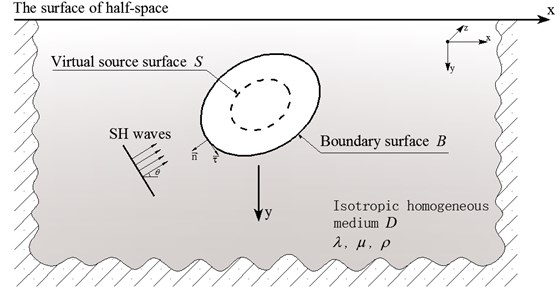Set $N$ observed points on boundary $B$, and $M$ points on the virtual sources $S$. We choose the unit center physical quantity instead of unit physical quantity, then the linear system of equations become:

9

Eq. (9) can be reformatted as:

10
$AX=b,$

where $A$ is the dense non-symmetric coefficients matrix, $X$ is the densities of fictitious sources on virtual boundary. $b$ denotes the response related to the free field on the boundary.

To speed up the calculation, a new algorithm of fast multi-pole fundamental solution method (FM-MFS) is presented. This method can greatly shorten the calculation time and reduce the needed memory. Combining General Minimize Residual Methods (GMRES)  with the fast multi-pole expansion technique , large-scale or high-frequency scattering of elastic waves can be solved effectively.

## 3. The new fast multi-pole for MFS

In traditional method of fundamental solution, it is difficult to calculate the approximate solutions for large-scale or high-frequency problems using the GMRES iterative algorithm. As shown in Fig. 2, consider a large number of cracks and inclusions existing in the actual complex terrain. For such problems, integration equations with high DOFs need to be calculated.

Fig. 2The calculation model of large-scale or multi-scatter problem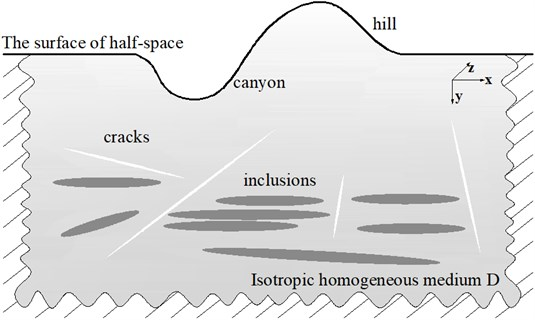To solve the Eq. (10), the large coefficients matrix $A$ is needed to storage. But the memory requirement is so high that this algorithm loses its practical value. The tree structure is used as the main storage and computing object in the FM-MFS, which can expand and transfer the kernel function. By taking the GMRES iterative algorithm, we can multiply $X$ and the tree structure instead of the large coefficients matrix $A$. Then results are obtained by controlling iterative precision. Different kernel functions apply to different expansion methods. In this paper, the plane wave expansion theorem is taken to expand the kernel function ${H}_{0}^{\left(2\right)}\left(•\right)$ in Eq. (7) as:

11
${H}_{0}^{\left(2\right)}\left(kr\right)=\frac{1}{\left(2p+1\right)}\sum _{n=0}^{2p}{R}_{n}\left(\stackrel{\to }{y{y}_{0}}\right)×{T}_{n}\left(\stackrel{\to }{{y}_{0}{x}_{0}}\right){S}_{n}\left(\stackrel{\to }{{x}_{0}x}\right),$
12
${R}_{n}\left(\stackrel{\to }{y{y}_{0}}\right)={e}^{ik\left({y}_{0}-y\right){\stackrel{^}{k}}_{n}},$
13
${S}_{n}\left(\stackrel{\to }{{x}_{0}x}\right)={e}^{ik\left(x-{x}_{0}\right){\stackrel{^}{k}}_{n}},$
14
${T}_{n}\left(\stackrel{\to }{{y}_{0}{x}_{0}}\right)=\sum _{m=0}^{p}{i}^{m}\left(2m+1\right){H}_{m}^{\left(2\right)}\left(kr\right){e}^{im\left(\phi -{\varphi }_{n}\right)},$

where, $\left|\stackrel{\to }{y{y}_{0}}\right|<\left|\stackrel{\to }{{y}_{0}{x}_{0}}\right|$ and $\left|\stackrel{\to }{{x}_{0}x}\right|<\left|\stackrel{\to }{{y}_{0}{x}_{0}}\right|\text{,}$${y}_{0}$is the center of multi-pole expansion, ${\stackrel{^}{k}}_{n}=\left(\mathrm{c}\mathrm{o}\mathrm{s}{\varphi }_{n},\mathrm{s}\mathrm{i}\mathrm{n}{\varphi }_{n}\right)\text{,}$ is the angle between $\stackrel{\to }{{y}_{0}{x}_{0}}$ and $X$ axis, $\phi =-\pi -\pi$, $r=\left|\stackrel{\to }{{y}_{0}{x}_{0}}\right|$, and the number $p$ of truncated.

Therefore, it can be shown that Eq. (6) can be rewritten as:

15
${\int }_{S}{\varphi }_{z}\left(y\right){T}_{k}\left(x,y\right)dS=\frac{i}{4\left(2p+1\right)}·\left[\sum _{n=0}^{2p}{M}_{n}\left(\stackrel{\to }{y{y}_{c}}\right)×\left(\frac{\partial {T}_{n}\left(\stackrel{\to }{{y}_{c}x}\right)}{\partial {n}_{x}}+\frac{\partial {T}_{n}\left(\stackrel{\to }{{y}_{c}x}\right)}{\partial {n}_{y}}\right)\right$

In which ${M}_{n}\left(\stackrel{\to }{y{y}_{c}}\right)$ and ${M}_{n}\left(\stackrel{\to }{{y}^{\mathrm{\text{'}}}{y}_{c}^{\mathrm{\text{'}}}}\right)$ are the multi-pole moments and can be expressed as:

16
${M}_{n}\left(\stackrel{\to }{y{y}_{c}}\right)={\int }_{S}{\varphi }_{z}\left(y\right){R}_{n}\left(\stackrel{\to }{y{y}_{c}}\right)dS,$
17
${M}_{n}\left(\stackrel{\to }{{y}^{\text{'}}{y}_{c}^{\text{'}}}\right)={\int }_{{S}^{\mathrm{\text{'}}}}{\varphi }_{z}\left(y\right){R}_{n}\left(\stackrel{\to }{{y}^{\text{'}}{y}_{c}^{\text{'}}}\right)d{S}^{\mathrm{\text{'}}}.$

${M}_{n}\left(\stackrel{\to }{y{y}_{c}}\right)$ and ${M}_{n}\left(\stackrel{\to }{{y}^{\text{'}}{y}_{c}^{\text{'}}}\right)$ in Eq. (15) are only related to ${y}_{c}$ and ${y}_{c}^{\text{'}}$. Furthermore, it needs to calculate only once for arbitrary collocation points $x$ for satisfying conditions.

Fig. 3Related points for FM-MFS expansion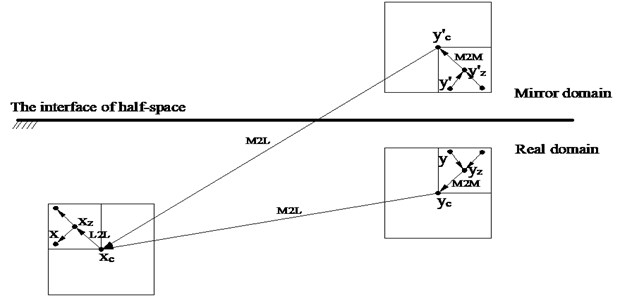As shown in Fig. 3, new multi-pole moments can be obtained when points for expansion moved from ${y}_{z}$, ${y\mathrm{\text{'}}}_{z}$ to ${y}_{c}$, ${y\mathrm{\text{'}}}_{c}$. The M2M transfer can be written as:

18
${M}_{n}\left(\stackrel{\to }{y{y}_{c}}\right)={e}^{ik\left({y}_{c}-{y}_{z}\right){\stackrel{^}{k}}_{n}}{M}_{n}\left(\stackrel{\to }{y{y}_{z}}\right),$
19
${M}_{n}\left(\stackrel{\to }{{y}^{\mathrm{\text{'}}}{y}_{c}^{\mathrm{\text{'}}}}\right)={e}^{ik\left({{y}^{\mathrm{\text{'}}}}_{c}-{{y}^{\mathrm{\text{'}}}}_{z}\right){\stackrel{^}{k}}_{n}}{M}_{n}\left(\stackrel{\to }{{y}^{\mathrm{\text{'}}}{y}_{z}^{\mathrm{\text{'}}}}\right).$

From equations above, the multi-pole moments do not need to be calculated again, but the calculated coefficient should be translated into new multi-pole moments.

Assuming a point ${x}_{c}$,which is close to point $x$, satisfying $\left|\stackrel{\to }{x{x}_{c}}\right|<\left|\stackrel{\to }{{y}_{c}{x}_{c}}\right|$. And the local expansion to Eq. (15) can be expressed as:

20
${\int }_{S}{\varphi }_{z}T\left(x,y\right)dS=\frac{i}{4\left(2p+1\right)}\sum _{n=0}^{2p}\left(\frac{\partial {S}_{n}\left(\stackrel{\to }{{x}_{c}x}\right)}{\partial {n}_{x}}+\frac{\partial {S}_{n}\left(\stackrel{\to }{{x}_{c}x}\right)}{\partial {n}_{y}}\right)×\left({L}_{n}\left(\stackrel{\to }{y{x}_{c}}\right)+{L}_{n}\left(\stackrel{\to }{{y}^{\text{'}}{x}_{c}}\right)\right),$

where, ${L}_{n}\left(\stackrel{\to }{y{x}_{c}}\right)$ and ${L}_{n}\left(\stackrel{\to }{{y}^{\mathrm{\text{'}}}{x}_{c}}\right)$ can be obtained by M2L transformation of multi-pole moments ${M}_{n}\left(\stackrel{\to }{y{y}_{c}}\right)$ and ${M}_{n}\left(\stackrel{\to }{{y}^{\mathrm{\text{'}}}{y}_{c}^{\mathrm{\text{'}}}}\right)$, as follows:

21
${L}_{n}\left(\stackrel{\to }{y{x}_{c}}\right)={T}_{n}\left(\stackrel{\to }{{y}_{c}{x}_{c}}\right){M}_{n}\left(\stackrel{\to }{y{y}_{c}}\right),$
22
${L}_{n}\left(\stackrel{\to }{{y}^{\text{'}{x}_{c}}}\right)={T}_{n}\left(\stackrel{\to }{{y}_{c}^{\text{'}}{x}_{c}}\right){M}_{n}\left(\stackrel{\to }{{y}^{\text{'}}{y}_{c}^{\text{'}}}\right).$

Finally, the local expansion transmission of L2L can be expressed as:

23
${S}_{n}\left(\stackrel{\to }{{x}_{c}x}\right)={e}^{ik\left({x}_{z}-{x}_{c}\right){\stackrel{^}{k}}_{n}}{S}_{n}\left(\stackrel{\to }{{x}_{z}x}\right).$

Take the scattering of SH waves by a cavity in a half space as an example, the implementation procedures is listed as follows.

Step 1. Discretization. Discrete the boundary $S$ and $B$, the same as in the T-MFS, and then automatically generate the adaptive tree structure (See Fig. 4).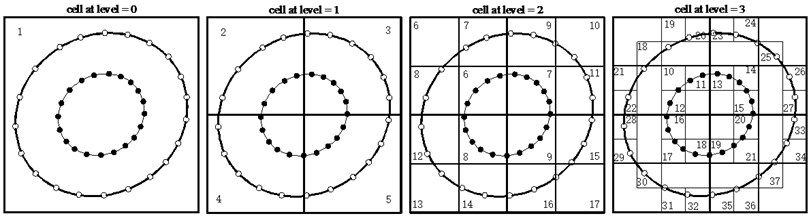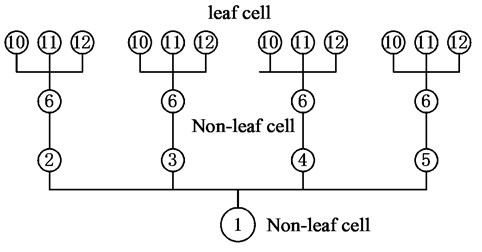b) Virtual wave source point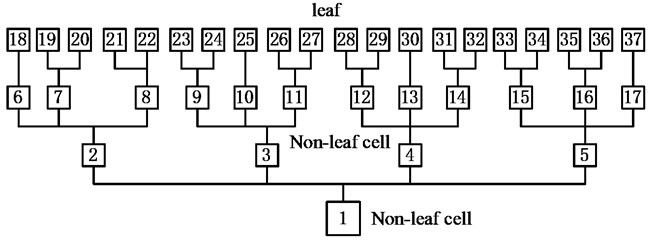c) Boundary point

Step 2. Upward pass. Translate information from the source points$y$, $y\mathrm{\text{'}}$ to the leaf cells. Eq. (16) and Eq. (17) are expanded in the center of leaves to generate multi-pole expansion moments. And then the multi-pole expansion point can be moved to the corresponding parent cell (M2M). Eq. (18) and Eq. (19) constitute the transfer relationship. In this step, transfer can be repeated several times to the parent cells. If satisfying the accuracy conditions, upward pass can be carried out ($l\ge$ 2) (see Fig. 4(a), (b)).

Step 3. Downward pass. The multi-pole expansion moments of each level cell on the tree structure can be transferred into corresponding local expansion moments (M2L), and Eq. (21) and Eq. (22) can be applied to build this relationship. Transfer the local expansion moments of each level cell in the tree structure to the leaf cell by using Eq. (23). So far, all local expansion moments at leaf cells can be translated to the boundary points $x$ through local expansion. The total expansion and transfer processes are completely given.

Step 4. Near-source calculation. The contributions of the near field virtual source points can be calculated by the T-MFS.

Step 5. Iterations. Update the unknown vector in the system $AX=b$ until the solution converges within a given tolerance using the GMRES solver.

## 4. Accuracy and computational efficiency

Taking the wave scattering around a cavity in half-space as an example, the accuracy and numerical stability of FM-MFS are tested and compared with analytical solution . As is shown in Fig. 1, assuming the radius of the circular hole is $a$, the number of collocation points on boundary $B$ is $N=$ 2000 ($M=N$), the embedment depth is $d=\text{2}a$, shear modular is $\mu$, surface width is $L=\text{8}a$, the incident angle of SH waves is $\theta =$ 90°,and the dimensionless frequency is $ka=$ 0-20, the expansion terms10, the iterative error is $\epsilon =$10-3. Fig. 5 illustrates the displacement amplitude on the surface by FM-MFS compared with that by analytical solution. Table 1 shows the displacement amplitude on the surface center for the vertical incident SH waves ($\theta =$ 90°) under different degrees of freedom. The comparison illustrates that the results by FM-MFS agree well with those by analytical solution, and have good numerical stability.

Fig. 5Comparisons between the displacement amplitude on the surface by FM-MFS and that of analytical solution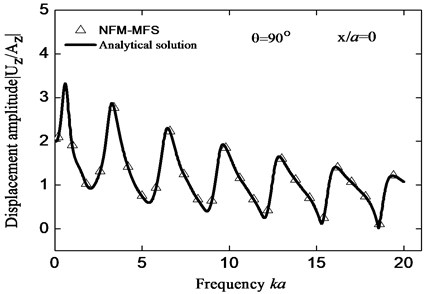Table 1Numerical stability of displacement amplitudes

 DOFs Displacement amplitude ($x/a=$ 0, $ka=\pi$) FM-MFS T-MFS 100 2.706531 2.706835 500 2.706502 2.706782 1000 2.706532 2.706774 5000 2.706553 2.706772 10000 2.706521 2.706772 Exact 2.706772 –

To test the efficiency of FM-MFS, the scattering of plane SH wave is considered by a cylindrical cavity in an elastic half space. Model parameters are as above and the DOFs increase from 100to 50000. As shown in Fig. 6, complexity of the T-MFS is $O\left({N}^{2}\right)$ about total CPU time, and the calculation time has reached 9147s when the DOFs are only 10000. However, the complexity of the FM-MFS is $O\left(N\right)$, and the calculation time is only 30 s when the DOFs reach 50000. Near field calculation becomes unnecessary due to low frequency, sources with small radius and the tree structure with fine mesh. Fig. 7 illustrates comparison of memory between T-MFS and the present FM-MFS. The storage requirement of T-MFS increases rapidly with the increase of DOFs. When the DOFs reaches 10000, the memory is more than 1.5 GB. However, the required memory will be greatly reduced when using the FM-MFS. For 50000 DOFs, the occupied memory is only 66 MB for the FM-MFS. Therefore, compared with the T-MFS, both the CPU time and memory performance are greatly improved by FM-MFS. These advantages speed up fast solution of 2D large-scale or broadband scattering problem of elastic waves.

Fig. 6Total CPU time used to solve the wave scattering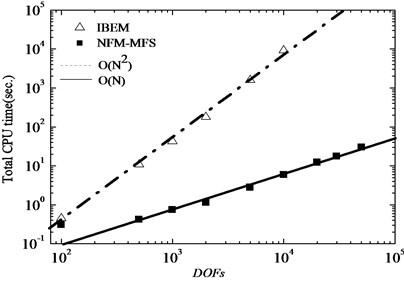Fig. 7Total memory used to solve the wave scattering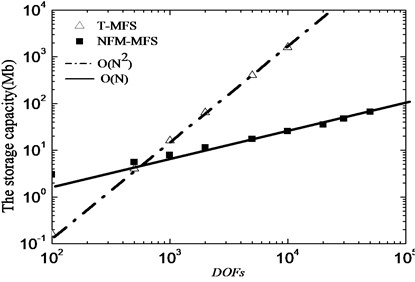Fig. 8The surface displacement amplitude for incident SH waves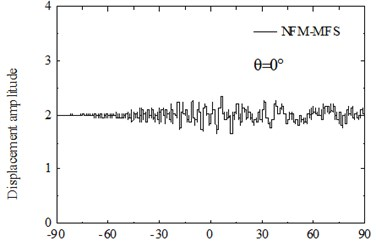a)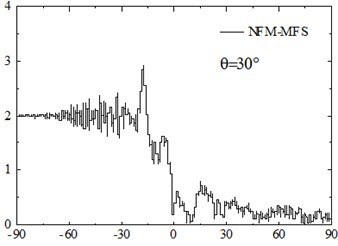b)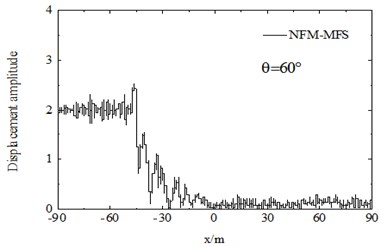c)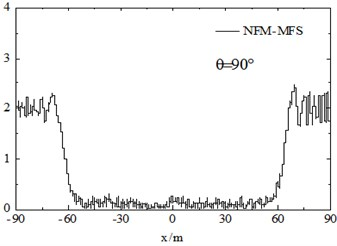d)

## 5. Numerical examples

### 5.1. The scattering of SH wave by cavities group

The FM-MFS can be used to calculate the scattering of seismic waves for shallow underground cavities distributed randomly in a half space. 30 m under the surface of the ground, 100 random cavities are established to simulate fracture within the scope of 60 m×120 m. Radiuses of circular cavities are ranging from 1.5 m to 3 m and 10,00 boundary points are set on the surface of each cavity. The total number of DOFs reaches 105. The dimensionless frequency is set as $ka=$ 150-300. The iterative error is $\epsilon =$ 10-3, and $\theta =$ 0°, 30°, 60°,90°. Fig. 8 shows the surface displacement amplitude of cavities. The consumed CPU time is 803 s and the storage of main matrix is 2011 MB.

Numerical results show that random cavities have significant effect on the scattering of SH waves. As shown in Fig. 8, for vertical incidence of the SH waves, the displacement amplitudes of the surface is greatly decreased, for example, the displacement amplitude near $x=$ 0 is only about 0.1. For the oblique incidence of SH waves, there is obvious amplification effect on the incident side and the amplitude close to two times of free-field displacement, but the displacement on the side behind the wave is significantly decreased. Therefore, random cavities group has a strong “barrier effect” on incident wave. Fig. 9 shows displacement amplitudes around the cavities under SH wave incidence. It can be seen that the coherence effect of scattering waves among cavities is very strong. The displacement amplitude reached more than 4.0 when the incident wave is close to the horizontal incidence. For the vertical incidence, the displacement amplitude around cavities is more than 1.5 times of the free field displacement amplitude. Therefore, for the safety of tunnel and underground pipeline engineering, crossing the cavities zone should be avoided, especially in the side of the incident wave.

Fig. 9The displacement amplitude around the cavities group for incident SH waves (ka= 150-300)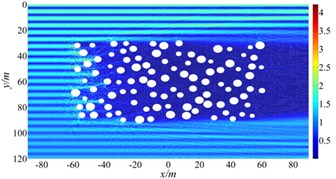a)$\theta =$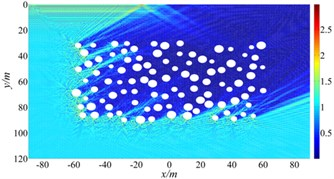b)$\theta =$ 30°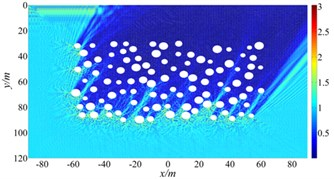c)$\theta =$ 60°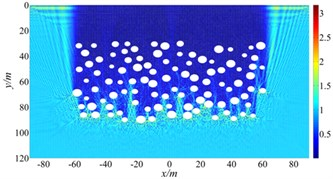d)$\theta =$ 90°

### 5.2. The scattering of SH wave by inclusions group

The scattering of plane SH wave is considered by inclusions group in a 30$a$×40$a$ domain, where $a$ is radius of the inclusion. Fig. 10 illustrates the results of displacement amplitude with variation of incident wave frequency. Calculation parameters are set to be: density ratio between the inclusion and the half space ${\rho }_{2}/{\rho }_{1}=$ 2/3, Poisson’s ratio ${\upsilon }_{1}=$ 0.25, ${\upsilon }_{2}=$ 0.30. DOFs = 61000. The tolerance for convergence is set to 10-3. In this calculation, the CPU time is about 205 seconds and the memory usage is about 1.3 GB, $ka=$ 0.5$\pi$, 1.0, 2.0, 5.0.

Assume incident plane waves propagating along $y$-direction. Fig. 10 plots the contours of displacement amplitude around inclusions group under SH wave incidence for $ka=$ 0.5$\pi$, 1.0, 2.0, 5.0, and shear-wave speed ratio ${\beta }_{2}/{\beta }_{1}=$ 1/2. It demonstrates that the scattering effect of elastic wave by inclusions seems more obvious as the frequency increases. For $ka=$ 1.0, the normalized displacement amplitude reaches up to 5.3, more than five times that of incident wave, which is mainly due to coherent effects of multiple scattering wave among inclusions group.

Fig. 10The displacement amplitude around the inclusions group for incident SH waves (θ= 0°)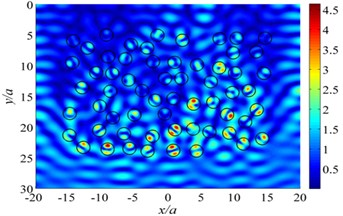a)$ka=$ 0.5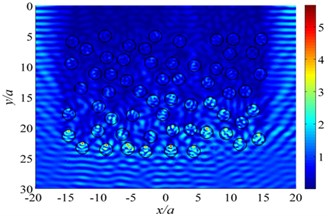b)$ka=$ 1.0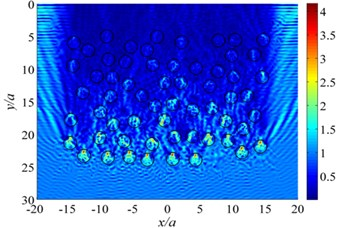c)$ka=$ 2.0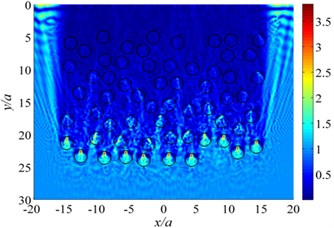d)$ka=$ 5.0

### 5.3. The scattering of SH wave by a V-shaped canyon

Displacement amplitude on the surface of a V-shaped canyon under incident high-frequency SH waves are shown in Fig. 12 and Fig. 13. The discrete number of canyon surface is $N=$ 200359, the calculation time is about 732 s, and the consumed memory is 2.4 GB. The parameters are set to be: the wideness of the V-shaped canyon 2 km, the incident frequency 20 Hz, the shear wave velocity 400 m/s, the dimensionless frequency $ka=$ 100$\pi$, $b$/$a=$ 1.0, 2.0, and the iterative error $\epsilon =$ 10-6, incident angle $\theta =$ 0°, 45°, 90° (Fig. 11).

Fig. 11Diffraction of plane SH waves by a V-shaped canyon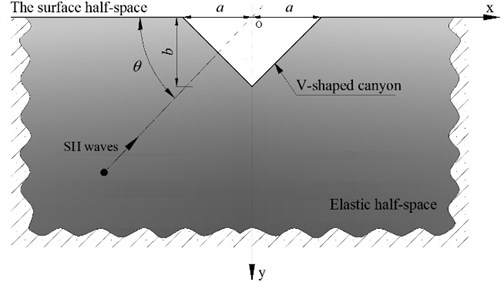Numerical results show that the left of the canyon appears significant displacement amplification effect with the incidence of high-frequency SH waves (left incidence), about twice the size of free-field displacement amplitude ratio. And the right side shows a very long “shadow zone”, indicating canyon has a shielding effect under the incident waves. Under oblique incidence, the amplification effect of canyon is particularly remarkable. With vertical incidence, the displacement amplitude at the corner of the V-shaped canyon is about 3.0, 50 % more than the free-field displacement. For high frequency wave incidence, the displacement response varies widely even in a small spatial scale, and displacement response of the canyon is violent on the side of the incident wave. Thus, to analyze the seismic response of bridge or dam located in canyon, the amplification effect of seismic waves scattering and uneven spatial distribution should be considered, and special attention should be paid to the high-frequency wave of oblique incidence. Calculation of the seismic responses of an engineering structure in a canyon may produce large error if using a uniform seismic input.

Fig. 12The surface displacement amplitude around a V-shaped canyon for incident SH waves (b/a= 1.0)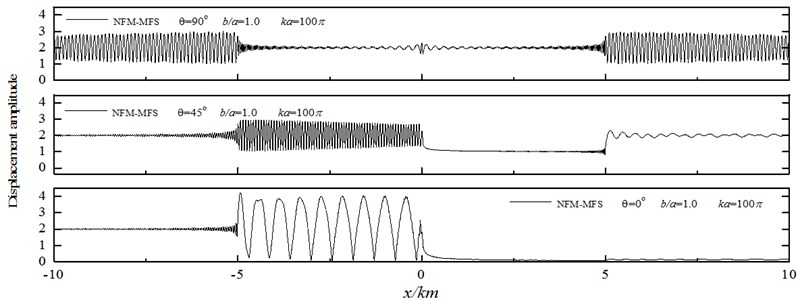Fig. 13The surface displacement amplitude around a V-shaped canyon for incident SH waves (b/a= 2.0)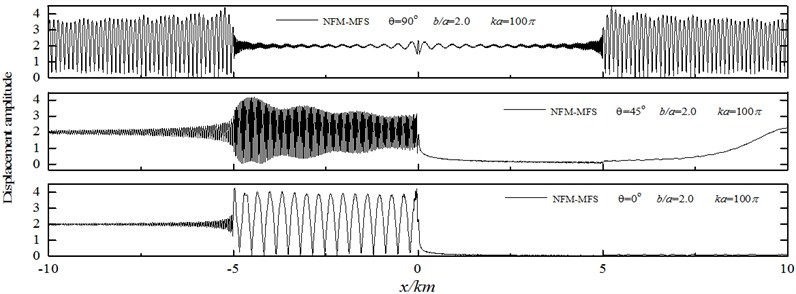### 5.4. The scattering and focusing of SH wave by a semi-elliptical hill

Fig. 14 illustrates the contour of displacement amplitude around a semi-elliptical hill under incident high-frequency SH waves. The parameters are set to be: the wideness of the hill 1km, the incident frequency 5 Hz, the shear wave velocity 500 m/s, the dimensionless frequency $ka=$ 10$\pi$, $b$/$a=$ 1.0, 2.0,5.0 and the iterative error $\epsilon =$ 10-6, incident angle $\theta =$ 60°, 90°.

The results show that the seismic response characteristics of the hill are significantly dependent on the incident wave angle and the aspect ratio of the hill. For the high steep hill, the focal effect of the seismic wave near the top is the most significant, such as for $b$/$a=$ 5.0, the displacement peak reaches 8.3, and the corresponding semi-circular hill displacement peak is 5.5. As for the spatial distribution feature, displacement amplification area is widely distributed on the semi-circular hill, but is mainly concentrated in the hill surface for the high steep hill. Thus, in actual violent earthquakes, the damage of high and steep slopes is usually serious. In addition, due to the coherence effect of the surface creeping wave, reflected wave and direct wave, the wave crest and wave node of the displacement response appear alternately on the surface of the hill, which is more significant for a high-steep hill. Considering the change of incident angle, the focusing position is also greatly shifted for oblique incidence, and the range of displacement amplification of a high and steep hill is enlarged. Correspondingly, the peak displacement is smaller than that of vertical incidence. The similar focusing phenomenon has also been observed by Chen et al. . Note that the accurate solution to the high-frequency wave scattering by a high-steep hill also illustrate excellent adaptability of the FM-MFS for treating practical problems.

Fig. 14The surface displacement amplitude around a semi-elliptical hill for incident SH waves (ka= 10π)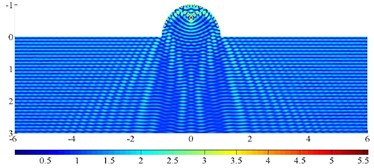a)$b$/$a=$ 1, $\theta =$90°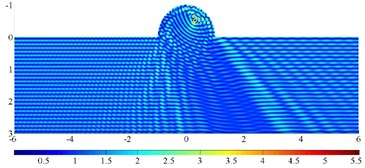b)$b$/$a=$ 1, $\theta =$ 60°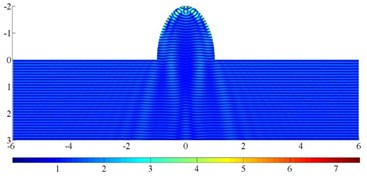c)$b$/$a=$ 2, $\theta =$ 90°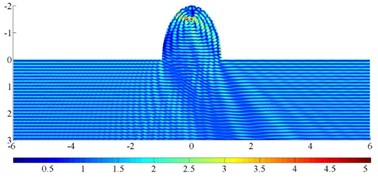d)$b$/$a=$ 2, $\theta =$ 60°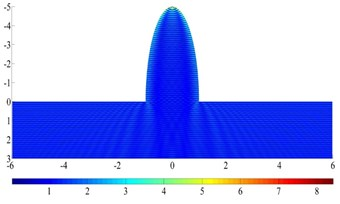e)$b$/$a=$ 5, $\theta =$ 90°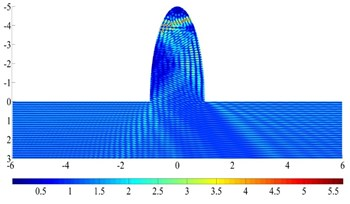f)$b$/$a=$ 5, $\theta =$ 60°

## 6. Conclusions

Combined with the new fast multi-pole expansion technique, the large-scale or high-frequency scattering problem of SH waves by 2-D superficial irregularities or heterogeneity in a solid half-space is solved by the proposed FM-MFS. By using the plane wave expansion of SH wave potential function, requirement of the CPU time and memory of multi-pole and local expansion is substantially reduced. The accuracy of this method is verified by comparing with exact solution. It is demonstrated that the FM-MFS can effectively solve practical complex scattering problem. Numerical results also show several interesting scattering characteristics of the high-frequency elastic waves generated by a V-shaped canyon, a semi-elliptical hill and inclusions (cavities) group. All these numerical examples indicate that the proposed FM-MFS has favorable numerical accuracy and efficiency, providing a novel effective method for the simulation of large-scale and high-frequency wave propagation problems for arbitrary-shaped scatters.

#### Acknowledgements

This work was supported in part by the National Natural Science Foundation of China (No. 51578372), and the Science and Technology Support Program Key Project of Tianjin (No. 17YFZCSF01140).

#### Cited by

Numerical simulation of anti-plane wave propagation in heterogeneous media
Amplification Effect of Ground Motion in Offshore Meandering Sedimentary Valley